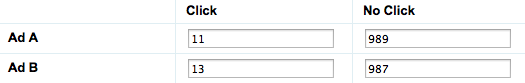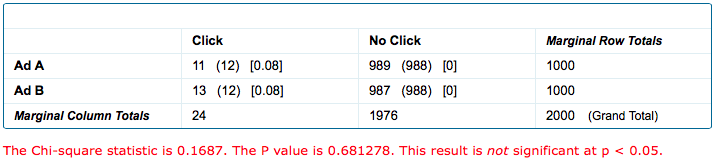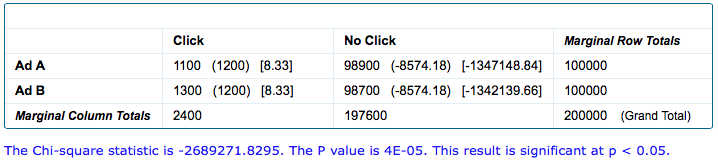# The Only* Statistical Significance Test You Need

July 01, 2014
By Jonathan Weber,
Director of Data PlatformsYou’ve heard the term “statistical significance”. But what does it really mean? I’m going to try to explain it as clearly and plainly as possible.

Suppose you run two different versions of an ad, and you want to know if the click-through rate was different (or you are comparing two different landing pages on bounce rate, or two campaigns on conversion rate). Ad A has a click-through rate of 1.1%, Ad B is 1.3%. Which one is better?

Seems like an easy answer: 1.3% > 1.1%, so Ad B is better, right? Well, not necessarily.

## Consider a quarterSuppose you have a quarter (and it’s a fair quarter, no tricks). The rate of getting heads when you flip should be 50%, right? If you flipped the coin an infinite number of times, you could expect it to come out heads half the time. Unfortunately in web analytics, we don’t have time to flip the quarter an infinite number of times. So maybe we only flip it 1000 times, and we get 505 heads and 495 tails. Do we conclude that heads are more likely than tails? What if we only flip it 100 times, or 10?

You can see that sometimes, the difference we measure is merely due to chance, not to a real difference.

## Statistical errors

When we measure something, there are two ways we could be wrong:

• There could be no difference between A and B, but we think we see a difference (false positive)
• There could be a real difference between A and B, but we fail to see it (false negative)

The significance level says, what’s the chance of making a false positive? That is, how likely is a difference we saw due merely to chance? This number is expressed as a probability (between 0 and 1, or a percentage between 0% and 100%) and is referred to by the letter p.

In our quarters example above with 1000 flips, p = 0.76, meaning there’s a 76% probability the difference was due merely to chance (we’ll see how to compute this in a minute). That’s pretty high! You get to decide what a low enough chance is to be comfortable with, but a common choice is p < 0.05, meaning there’s less than 5% chance we’re wrong (or we’re wrong less than about 1 time in 20). Given that standard, we would say the difference in the rate of heads and tails is “not statistically significant”.

So, statistical significance is related to the chance of a false positive. What about false negatives? There’s a related concept called the statistical power or sensitivity, which can help you estimate up front how large a sample size you need to detect a difference (how many times you need to flip the coin). Statistical power is a bit more complicated, so we’ll save it for another time.

## Doing the calculation: the chi-squared testOK, so how can we find the p-value for our ad test? We can use a statistical test called “Pearson’s chi-squared (χ²) test” or the “chi-squared test of independence” or just “chi-squared test”. Don’t worry; you don’t actually have to know any fancy math or Greek letters to do this. (To sound smart, you should know that “chi” rhymes with pie and starts with a “k”. Not like Chi-Chis, the sadly defunct Mexican restaurant.)

The chi-squared test only applies to a categorical variable (yes/no or true/false, for example), not to a continous variable (a number). Although you might look at your web analytics and say, “I have all numbers!”, in fact many of your metrics are hidden categorical variables. Click-through rate is just a percentage measurement for a yes/no situation: they clicked or they didn’t. Bounce rate: they bounced or they didn’t. Conversion rate: they converted or they didn’t. And conveniently, those are probably three of the most common metrics you’d want to test.

To perform the chi-squared test, you need the number of successes and failures for each variation. This makes what’s called a contingency table, something like this:Here’s an easy web page where you can fill in your categories and numbers and it will calculate the chi-squared test and give you the p-value. (Remember, lower is better for the p-value, and you should pick some threshold like p < 0.05 that you are going to consider as significant.)

You can also do this in Excel using the CHISQ.TEST function, should you need to.

Either way, the results you get will be something like this:The “chi-squared statistic” and “degrees of freedom” are just the values the fancy (#notthatfancy) math of the test uses to calculate the part we really care about: the p-value. In this case, you can see that if my ad test was based on only 1000 impressions for each of Ad A and Ad B, p = 0.68. Not significant at the p < 0.05 level.

If my ad test was instead based on 100,000 impressions for each of Ad A and Ad B, p = 0.00004. Definitely significant at the p < 0.05 level! The number of times we flipped the coin makes a big difference.## Be careful of repeated comparisons

Remember, p = 0.05 would mean we’d see a difference where there actually isn’t 1 time out of 20, on average. You need to be careful of this if you do repeated comparisons on the same data. For example, suppose we throw Ad C into the mix in addition to Ad A and Ad B. Now we can make 3 comparisons: A vs. B, A vs. C, and B vs. C. But we’ve also compounded the chances we’ve made an error. If we made 20 comparisons, we’d likely be wrong on one of them. (That’s slightly simplistic, since it depends on whether there really are differences between the ads and how big the differences are, but you get the idea.)

You can take care of this with the fancy sounding “Bonferroni correction”, which is actually very simple. It says this: if you are making n comparisons, divide your p-value threshold by n. So if we were looking for p < 0.05 but we were making 3 comparisons, we’d divide 0.05 / 3 = 0.017, using that as our threshold for significance.

## *Really the only test I need to know?

OK, so no. The chi-square test only works for categorical variables, not for continuous variables like pages per session or time on site. So for variables like those, you probably need a t-test instead (we’ll save that for a future installment).And, if you get enough statisticians together in a room, they will tell you about all sorts of ways your chi-squared test is inadequate. You should use the Yates correction, or a G-test, or Fisher’s exact test. You need McNemar’s test for repeated observations on the same subjects.

To this I say: yeah, yeah, yeah. Fisher’s exact test is better, but really only matters for small sample sizes (like, say, 20). What are you doing testing ads with only 20 impressions? We’re not looking for cancer in rats here. You’re wasting your time. Repeated observation with the same subjects? Again, maybe in a clinical trial, not so much in web analytics. Your chi-squared test is just fine, plus it has the advantage of being widely known, used, and understood, so you can communicate the results to others without spending time haggling over your statistical methods and just get to the point.

In any case, I hope this has been an illuminating look at statistical significance, so that you can finally start to compare those numbers in your web analytics and say, are these really different?